## 17 Oct Arrays in C Programming

An array is a collection of elements, of fixed size and type, for example, a collection of 5 elements of type int. It is a data structure to group elements. In this lesson, we will learn how to work with arrays in C Language.

Let’s see another example to understand the concept.

A class has 20 students and you want to arrange the marks of all the 20 students. You can do this using the following two methods,

Method 1
Declare 20 different variables for each student’s marks, with their own identifier. That would be cumbersome, right?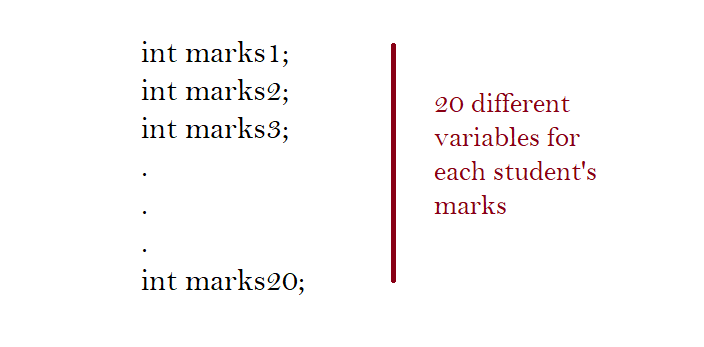Method 2
Declare a single array variable and store the 20 different values (marks) in contiguous memory locations i.e. use one-dimensional arrays.

You saw two methods above. Definitely, Method 2 is better, since you do not need to declare different variables for the same type of elements.

Accordingly, method 2 would look like the following,

##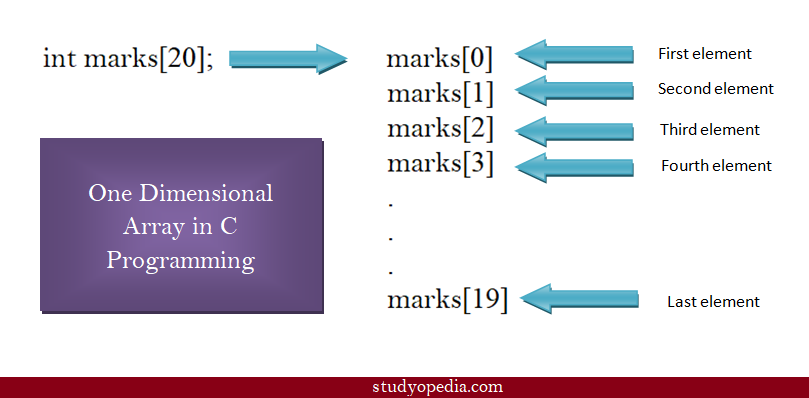Above you saw how arrays work in C Language. For individual array elements, we use the index under square brackets. Index 0 is for the first element, Index 1 is for the second element and it goes on. This means the first element has an index of 0, and the last element is 19 for our 20 elements array for student marks. These terms would be new to you since you just begin learning arrays.

Now, we will get into more details about array declaration and initialization.

## How to declare arrays in C Language

For array declaration in C Programming, you need to specify the datatype and the count of elements you want the array to be. Here, we are discussing about one-dimensional arrays. For example, write , if you want to declare an array with 3 elements.

The following syntax will make the concept clearer,

Here,
datatype: C data type such as int, float, etc.
name_of_array: The name of an array (variable)
size_of_array: The size of the array under square brackets. Add a value greater than zero.

The following example shows the perfect way of declaring arrays in C,

Above, we declared an array, with datatype int, name of array marks, with 3 elements, since the size we specified is 3. The variable array marks now hold 3 elements.

## How to initialize arrays

To initialize arrays, you need to add the array values in a single line. Continuing the previous example,

To initialize the above, add the array values like this in braces,

Above, we added marks for 3 students by initializing our array. Always remember, element one would come at index 0 and the last element at size – 1.

For example,

## First C Program with Arrays

To move further, let us see a complete C Program, and learn how to declare and initialize arrays. We will also see how to access array elements,

The following is the output, displaying declaration, initialization of one-dimensional array, and how to access individual array elements,## Two Dimensional Arrays in C

An array can have two or more dimensions. Here we will discuss two-dimensional arrays. A two-dimensional array as the name suggests has two dimensions, not one like we saw in one-dimensional arrays.

Before the example, let’s see the syntax,

Considering the above syntax, let’s see an example, with 3 rows and columns in a two-dimensional array,

Let’s now see how rows and columns work in a two-dimensional array,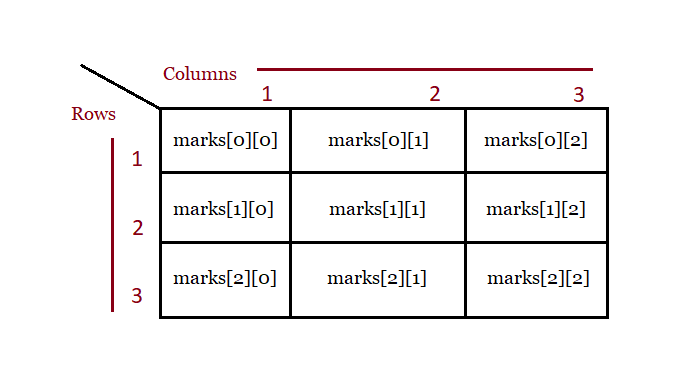### Example

Let’s see a program where we will print the rank and points of 5 cricketers. Here, you can see an array with rows and columns i.e. two-dimensional.

We’re displaying the rank and points for 5 teams, but before that, you need to input the values. %d is a format specifier and % is a placeholder. Specifying %d means displaying values as integers, so we’ve used it.

Here, cric would mean 1st row and 1st column, cric is 1st row and 2nd column, etc

Following is the output,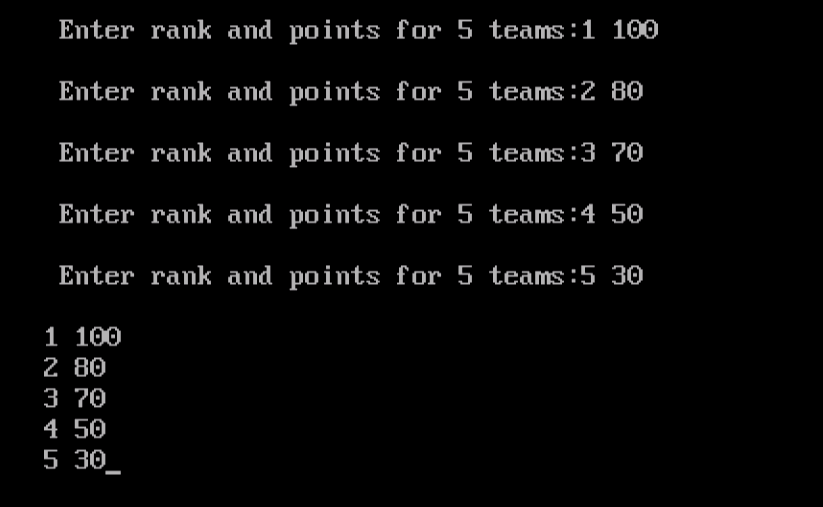Above, we saw the following two-dimensional arrays,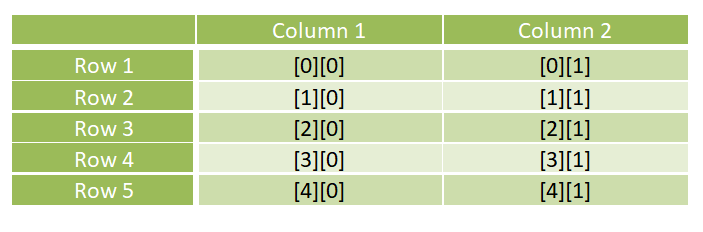If you liked the tutorial, spread the word and share the link and our website Studyopedia with others: